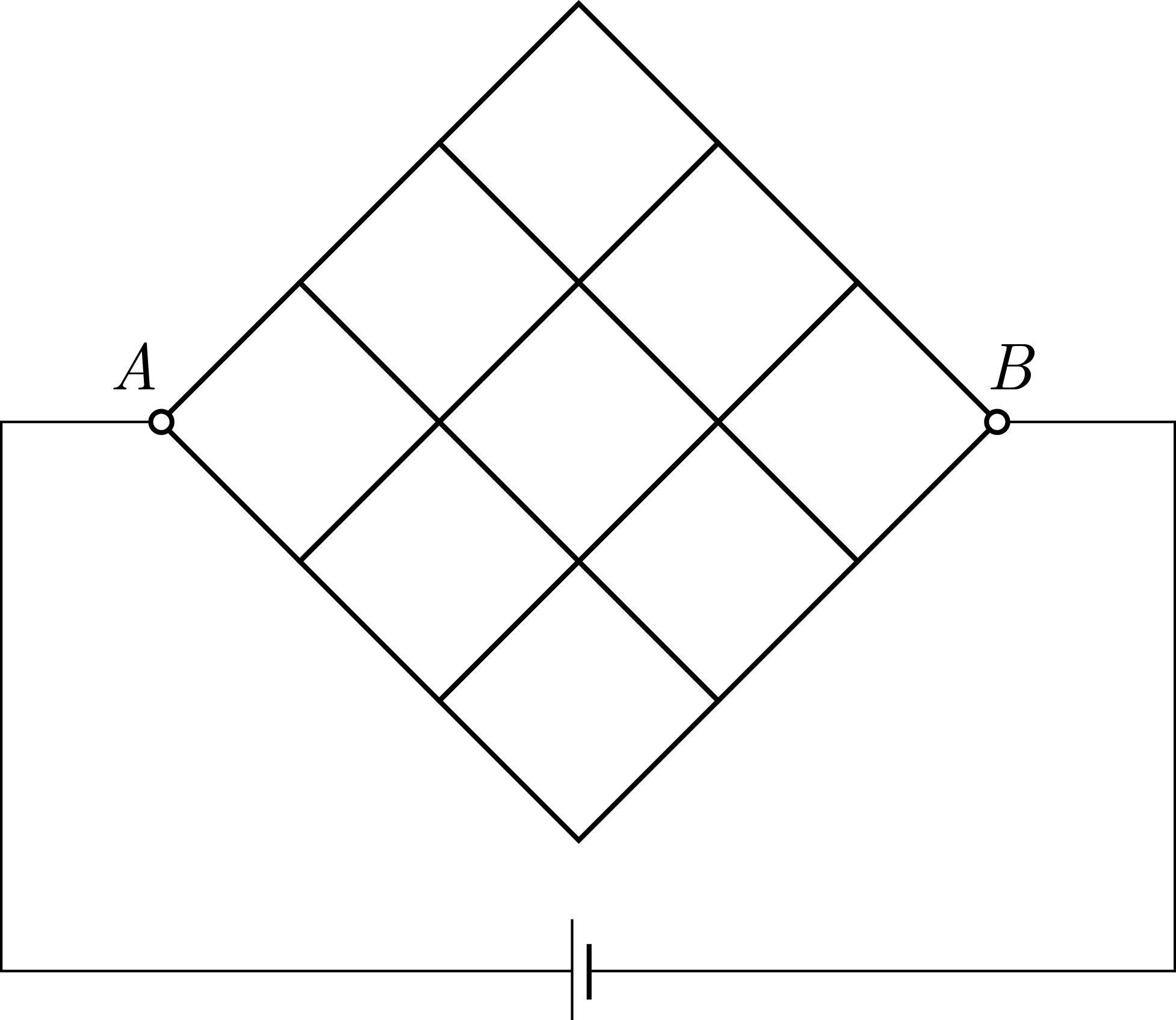Resistors!As seen above, a set of resistors are arranged in forms of the cells, each side having a resistance $R$. If $R_0$ represents between the two points $A$ and $B$. If $\dfrac{R_0}R = \dfrac ab$ for coprime positive integers, find the value of

$a+b+ab.$

×